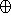# Planar Minkowski SumsReference Manual

Ron Wein

## Introduction

This package consist of functions for computing the Minkowksi sum of two polygons in the plane. Namely, given two polygons P,Q2 it computes PQ = { p + q  |  pP, qQ }.

In addition, the package also includes functions for offsetting a polygon, namely computing its Minkowski sum with a disc of a given radius. It is possible to compute the exact representation of the offset, or to approximate it with guaranteed error bounds, in order to speed up the computation time.

### Classes

CGAL::Small_side_angle_bisector_decomposition_2<Kernel,Container>
CGAL::Optimal_convex_decomposition_2<Kernel,Container>
CGAL::Hertel_Mehlhorn_convex_decomposition_2<Kernel,Container>
CGAL::Greene_convex_decomposition_2<Kernel,Container>